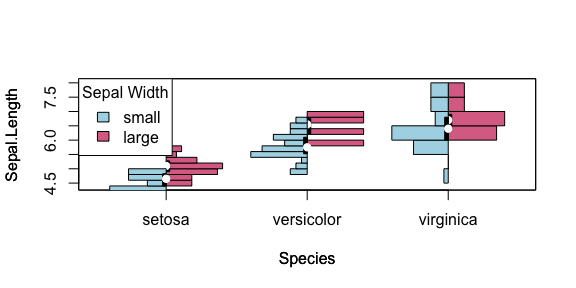# Customising Histogram Plots with Formula Input

#### 2022-12-09

Since boxplots have become the de facto standard for plotting the distribution of data most users are familiar with these and the formula input for dataframes. However this input is not available in the standard histoplot package. Thus it has been restored here for enhanced backwards compatibility with boxplot.

As shown below for the iris dataset, histogram plots show distribution information taking formula input that boxplot implements but histoplot is unable to. This demonstrates the customisation demonstrated in the main histoplot vignette using histoplot syntax with the formula method commonly used for boxplot, t.test, and lm.

library("vioplot")
data(iris)
boxplot(Sepal.Length~Species, data = iris)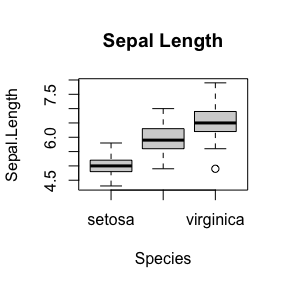Whereas performing the same function does not work with vioplot (0.2).

devtools::install_version("vioplot", version = "0.2")
library("vioplot")
vioplot(Sepal.Length~Species, data = iris)
Error in min(data) : invalid 'type' (language) of argument

## Plot Defaults

vioplot(Sepal.Length~Species, data = iris)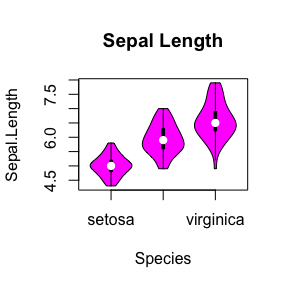Another concern we see here is that the vioplot defaults are not aesthetically pleasing, with a rather glaring colour scheme unsuitable for professional or academic usage. Thus the plot default colours have been changed as shown here:

vioplot(Sepal.Length~Species, data = iris, main = "Sepal Length")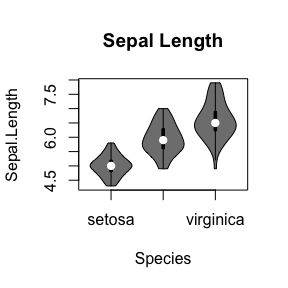## Plot colours: Histogram Fill

Plot colours can be further customised as with the original vioplot package using the col argument:

histoplot(Sepal.Length~Species, data = iris, main = "Sepal Length", col="lightblue")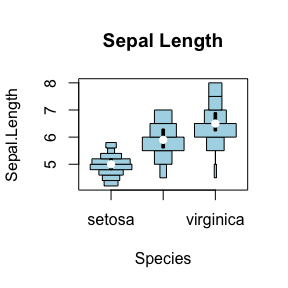### Vectorisation

However the vioplot (0.2) function is unable to colour each histogram separately, thus this is enabled with a vectorised col in histoplot (0.4):

histoplot(Sepal.Length~Species, data = iris, main = "Sepal Length", col=c("lightgreen", "lightblue", "palevioletred"))
legend("topleft", legend=c("setosa", "versicolor", "virginica"), fill=c("lightgreen", "lightblue", "palevioletred"), cex = 0.5)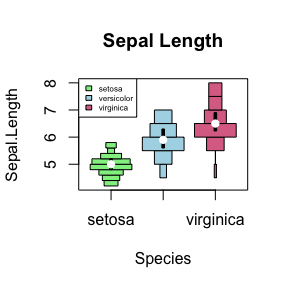## Plot colours: Violin Lines and Boxplot

Colours can also be customised for the histogram fill and border separately using the col and border arguments:

histoplot(Sepal.Length~Species, data = iris, main = "Sepal Length", col="lightblue", border="royalblue")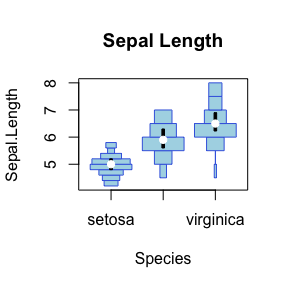Similarly, the arguments lineCol and rectCol specify the colours of the boxplot outline and rectangle fill. For simplicity the box and whiskers of the boxplot will always have the same colour.

histoplot(Sepal.Length~Species, data = iris, main = "Sepal Length", rectCol="palevioletred", lineCol="violetred")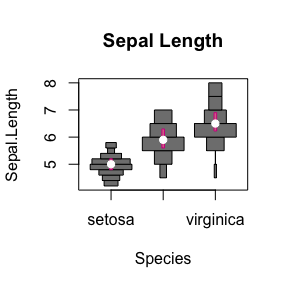The same applies to the colour of the median point with colMed:

histoplot(Sepal.Length~Species, data = iris, main = "Sepal Length", colMed="violet")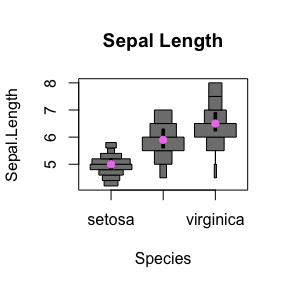### Combined customisation

These can be customised colours can be combined:

histoplot(Sepal.Length~Species, data = iris, main = "Sepal Length", col="lightblue", border="royalblue", rectCol="palevioletred", lineCol="violetred", colMed="violet")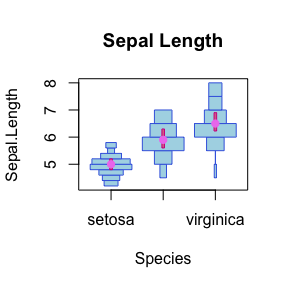### Vectorisation

These colour and shape settings can also be customised separately for each histogram:

histoplot(Sepal.Length~Species, data = iris, main="Sepal Length", col=c("lightgreen", "lightblue", "palevioletred"), border=c("darkolivegreen4", "royalblue4", "violetred4"), rectCol=c("forestgreen", "blue", "palevioletred3"), lineCol=c("darkolivegreen", "royalblue", "violetred4"), colMed=c("green", "cyan", "magenta"), pchMed=c(15, 17, 19))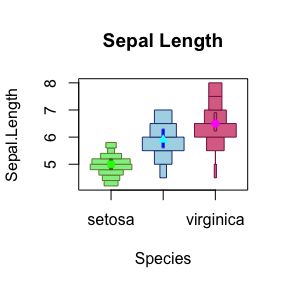## Split Bihistogram Plots

We set up the data with two categories (Sepal Width) as follows:

data(iris)
summary(iris$Sepal.Width) ## Min. 1st Qu. Median Mean 3rd Qu. Max. ## 2.000 2.800 3.000 3.057 3.300 4.400 table(iris$Sepal.Width > mean(iris$Sepal.Width)) ## ## FALSE TRUE ## 83 67 iris_large <- iris[iris$Sepal.Width > mean(iris$Sepal.Width), ] iris_small <- iris[iris$Sepal.Width <= mean(iris\$Sepal.Width), ]

A direct comparision of 2 datasets can be made with the side argument and add = TRUE on the second plot:

histoplot(Sepal.Length~Species, data=iris_large, col = "palevioletred", plotCentre = "line", side = "right")
histoplot(Sepal.Length~Species, data=iris_small, col = "lightblue", plotCentre = "line", side = "left", add = T)
title(xlab = "Species", ylab = "Sepal Length")
legend("topleft", fill = c("lightblue", "palevioletred"), legend = c("small", "large"), title = "Sepal Width")